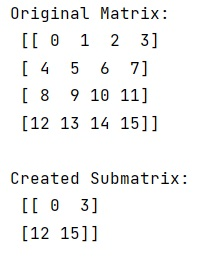# NumPy: Extract Submatrix

Given a NumPy matrix, we have to extract a submatrix from it.
Submitted by Pranit Sharma, on January 23, 2023

NumPy is an abbreviated form of Numerical Python. It is used for different types of scientific operations in python. Numpy is a vast library in python which is used for almost every kind of scientific or mathematical operation. It is itself an array which is a collection of various methods and functions for processing the arrays.

A matrix that contains certain rows and columns of a larger matrix is known as a submatrix.

## Problem statement

Suppose that we are given a defined row and column structured matrix and we need to find a way to extract a submatrix from this matrix.

## Extracting Submatrix from a NumPy Matrix

To extract a submatrix, we will use numpy.ix_() method. This method constructs an open mesh from multiple sequences.

This function takes N 1-D sequences and returns N outputs with N dimensions each, such that the shape is 1 in all but one dimension, and the dimension with the non-unit shape value cycles through all N dimensions.

Let us understand with the help of an example,

## Python program to extract submatrix from a NumPy matrix

```# Import numpy
import numpy as np

# Creating a numpy array
arr = np.arange(16).reshape(4,4)

# Display original array
print("Original Matrix:\n",arr,"\n")

# Creating a submatrix
res = arr[np.ix_([0,3],[0,3])]

# Display result
print("Created Submatrix:\n",res,"\n")
```

## Output

The output of the above program will be: